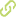# The metric dimension of the circulant graph c(n, ±{1, 2, 3, 4}) Journalartikel### Abstract

• Let G =(V,E) be a connected graph and let d(u, v) denote the distance between vertices u, v ∈ V. A metric basis for G is a set B ⊆ V of minimum cardinality such that no two vertices of G have the same distances to all points of B. The cardinality of a metric basis of G is called the metric dimension of G, denoted by dim(G). In this paper we determine the metric dimension of the circulant graphs C(n, ±{1, 2, 3, 4}) for all values of n.

• 2017

### Review-Status

• Peer-Reviewed

• Open Access

• 3

• 69

• 417

• 441